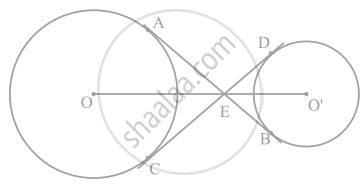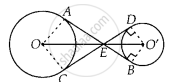# In figure the common tangent, AB and CD to two circles with centres O and O' intersect at E. Prove that the points O, E, O' are collinear. - Mathematics

Sum

In figure the common tangent, AB and CD to two circles with centres O and O' intersect at E. Prove that the points O, E, O' are collinear.#### Solution

Join AO, OC and O’D, O’B.

Now, in ∆EO’D and ∆EO’B,

O’D = O’B

O’E = O’E

ED = EB  .......[Tangents drawn from an external point to the circle are equal in length∴ EO’D ≅ ∆ EO’B   .......[By SSS congruence criterion]

⇒ ∠O’ED = ∠O’EB   ......(i)

i.e., O’E is the angle bisector of ∠DEB.

Similarly, OE is the angle bisector of ∠AEC.

∠O’DE = ∠O’BE = 90°  .......[CED is a tangent to the circle and O’D is the radius, i.e., O’D ⊥ CED]

⇒ ∠O’DE + ∠O’BE = 180°

∴ ∠DEB + ∠DO’B = 180°  .......[∵ DEBO’ is cyclic quadrilateral]  .......(ii)

Since, AB is a straight line.

∴ ∠AED + ∠DEB = 180°

⇒ ∠AED + 180° – ∠DO’B = 180°   .....[From (ii)]

⇒ ∠AED = ∠DO’B   ......(iii)

Similarly, ∠AED = ∠AOC  .......(iv)

Again from eq. (ii), ∠DEB = 180° – ∠DO’B

Dividing by 2 on both sides, we get

1/2 ∠DEB = 90^circ - 1/2 ∠DO'B

⇒ ∠DE'O = 90° - 1/2 ∠DO'B    ......(v) [∵ O'E is the angle bisector of ∠DEB i.e. 1/2 ∠DEB = ∠DEO']

Similarly, ∠AEC = 180° – ∠AOC

Dividing vy 2 on both sides, we get

1/2 ∠AEC = 90^circ - 1/2 ∠AOC  .....(vi) [∵ OE is the angle bisector of ∠AEC i.e., 1/2 ∠AEC = ∠AEO]

Now, ∠AED + ∠DEO' + ∠AEO = ∠AED + (90^circ - 1/2 ∠DO"'"B) + (90^circ - 1/2 ∠AOC)

= ∠AED + 180^circ - 1/2 (∠DO"'"B + ∠AOC)

= ∠AED + 180^circ - 1/2 (∠AED + ∠AED)  .....[From equation (iii) and (iv)]

= ∠AED + 180^circ - 1/2 (2 xx ∠AED)

= ∠AED + 180° – ∠AED = 180°

∴ ∠AED + ∠DEO' + ∠AED = 180°

So, OEO’ is straight line.

Hence, O, E and O’ are collinear.

Concept: Tangent to a Circle
Is there an error in this question or solution?

#### APPEARS IN

NCERT Mathematics Exemplar Class 10
Chapter 9 Circles
Exercise 9.4 | Q 10 | Page 111
Share